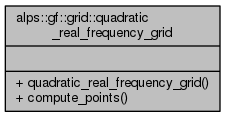ALPSCore reference
alps::gf::grid::quadratic_real_frequency_grid Class Reference

Quadratic grid in real frequency. More...

#include <grid.hpp>

Collaboration diagram for alps::gf::grid::quadratic_real_frequency_grid:## Public Member Functions

quadratic_real_frequency_grid (double spread, int n)

void compute_points (std::vector< double > &points) const

## Detailed Description

Quadratic grid in real frequency.

Definition at line 80 of file grid.hpp.

## Constructor & Destructor Documentation

 alps::gf::grid::quadratic_real_frequency_grid::quadratic_real_frequency_grid ( double spread, int n )
inline

Definition at line 86 of file grid.hpp.

## Member Function Documentation

 void alps::gf::grid::quadratic_real_frequency_grid::compute_points ( std::vector< double > & points ) const
inline

Definition at line 91 of file grid.hpp.

The documentation for this class was generated from the following file: